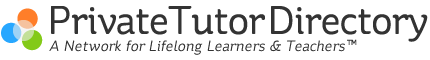How to do a formal geometric proof.
December 16, 2008 by Joe Perlman  |

Every teacher has a their own method for doing a formal proof. Some tell you to put all the givens in at one shot then work with the picture and the givens to make a proof. A good suggestion is to systematically use the givens! For example, if you are given:

Given: E is the midpoint of DF, and BE is perependicular to DF..........

What we can do is use each given like a wrapped present. Take the given and unwrap it until it is a congruent sign. Otherwise said, we can pretend to have a math conversation. So in this case, we would start with E is the midpoint of DF. If we were having a conversation at the local restaurant, I would say so what does that mean? You would then answer, "Then DE = FE" and I would say why is that? Your reason is the definition of a midpoint.

So in your table I would see

1) E is the Midpoint of DF   1) Given

2) DE = FE                        2) Def. of midpoint

Once you have a congruent sign, move onto the next given. So you would say that BE is perpendicular to DF. I would say why, you would say given. Then I would ask what does that mean and you would say that means that BED and BEF are right angles. I would ask why and you would say because it is the definition of perpendicular lines. I would say what does that mean then, and you would say that BED and BEF are congruent. I would say why and you would say that all right angles are congruent. In a proof it looks like

3) BE is perp DF                3) Given

4)

5)

This can keep going for a while - if you have more questions - just email. I have a very high rate of students with fabulous scores on geometry proofs.